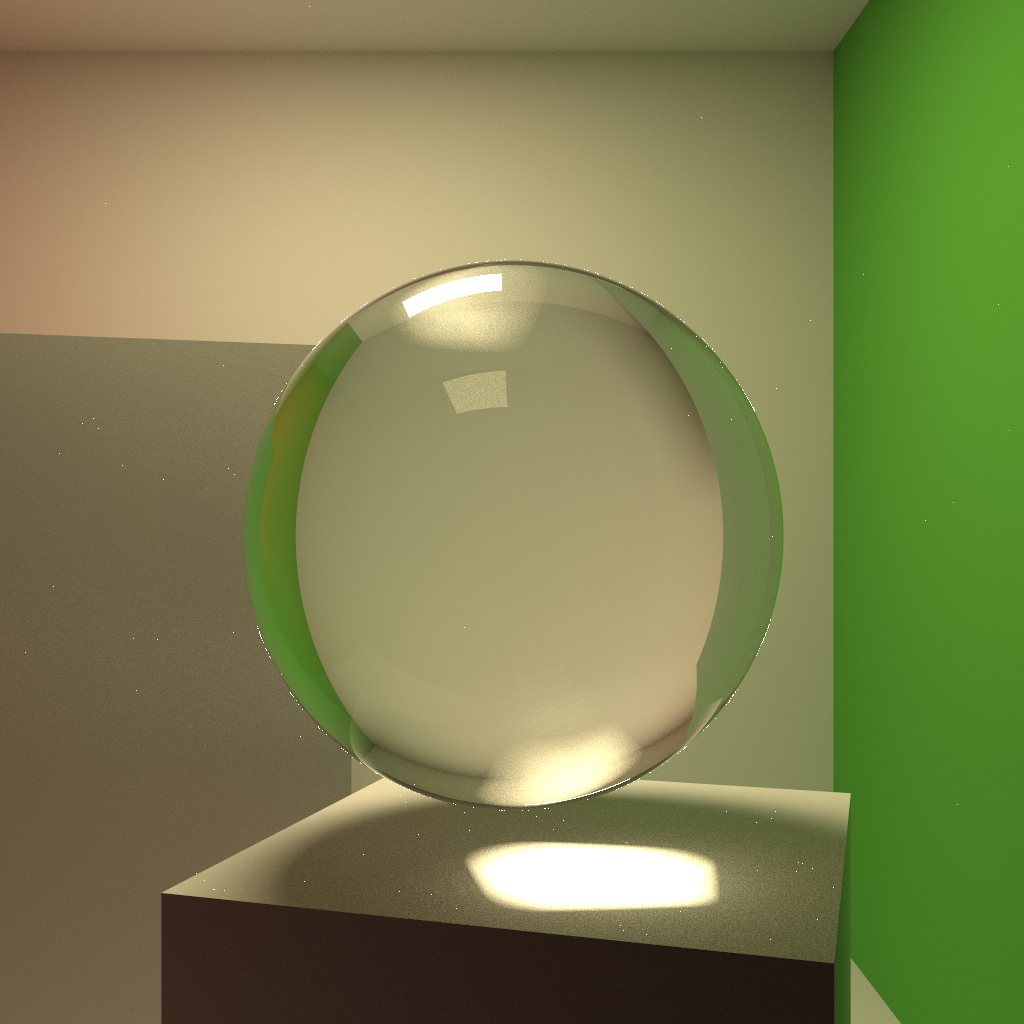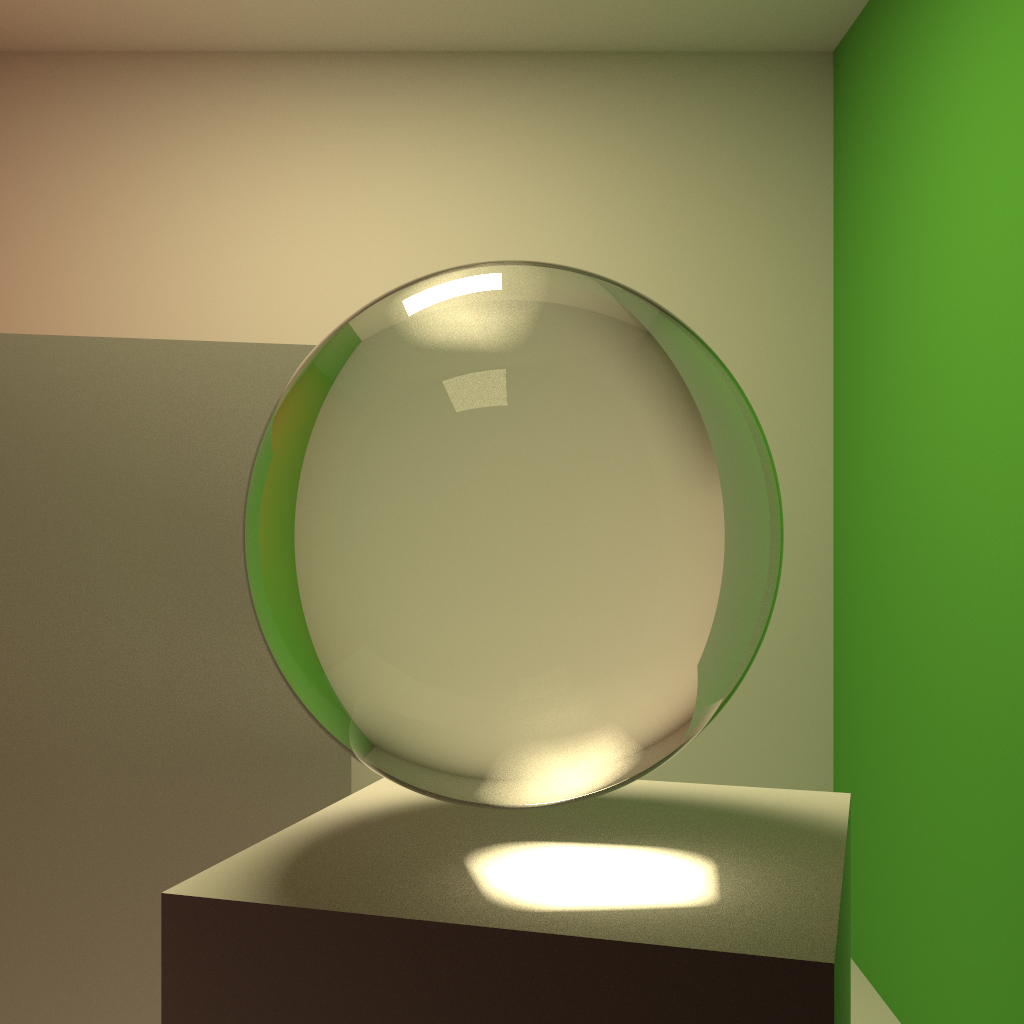## Consistent Normal Interpolation

I recently ran into a problem with interpolated normals. Instead of supporting sphere primitives directly, Takua Rev 5 generates polygon mesh spheres and handles them the same way as any other polygon mesh is handled. However, when I ran a test using a glass sphere, a lot of fireflies appeared:The fireflies are an artifact arising from how normal interpolation interacts with specular materials. Since the sphere is a polygonal mesh, normal interpolation is required to give the sphere a smooth appearance instead of a faceted one. The interpolation scheme I was using vanilla Phong normal interpolation: store a smoothed normal at each vertex, and then calculate the smooth shading normal at each point as the barycentric-coordinate-weighted sum of the smooth normals at each vertex of the current triangle. This works well for most cases, but a problem arises at grazing angles: since the smooth shading normal corresponds not to the actual geometry but to a “virtual” smoothed version of the geometry, sometimes outgoing specular rays will end up going below the tangent plane of the current hit point. Because of this, rays hitting a glass sphere with Phone normal interpolation at a grazing angle can sometimes go the wrong way, hence the artifacts in the above image.

Of course, the closer the actual geometry lines up to the virtual smoothed geometry, the less this grazing angle problem occurs. However, in order to completely eliminate artifacting, the polygon geometry needs to approach the limit of the virtual smoothed geometry. In this render, I regenerated the sphere with two more levels of subdivision. As a result, there are fewer fireflies, but fireflies are still present:Initially I thought about just getting rid of the fireflies by checking pixel intensities and clamping intensities that were significantly brighter than their immediate neighbors, which is a fairly basic/standard firefly reduction strategy. However, since in this case the fireflies occur mostly at grazing angles and therefore on silhouettes, intensity clamping can lead to some unpleasant aliasing on silhouettes.

Fortunately, there was a paper by Alexander Reshetov, Alexei Soupikov, and William R. Mark at SIGGRAPH Asia 2010 about dealing with this exact problem. The paper, “Consistent Normal Interpolation”, presents a simple method for tweaking Phong normal interpolation to guarantee that reflected rays never go below the tangent plane. The method is based on incoming ray direction and the angle between the smooth interpolated normal and true face normal. The actual method presented in the paper is very straightforward to implement, but the derivation of the algorithm is fairly interesting and involves solving a nontrivial optimization problem to find a scaling term.

I implemented a slightly modified version of the algorithm presented on page 5 of the paper. The modification I made is simply to account for rays hitting polygons from below the tangent plane, as in the case of internal refraction. Now interpolated normals at grazing angles no longer produce firefly artifacts:I’m working on writing up a lot of stuff, so more soon! Stay tuned!